# Иностранная литература и современность: General Statistics: Ch 5 HW Flashcards

Set Details Share
created 8 years ago by GreenHero64
180,726 views
This course is designed to acquaint the student with the principles of descriptive and inferential statistics. Topics will include: types of data, frequency distributions and histograms, measures of central tendency, measures of variation, probability, probability distributions including binomial, normal probability and student's t distributions, standard scores, confidence intervals, hypothesis testing, correlation, and linear regression analysis. This course is open to any student interested in general statistics and it will include applications pertaining to students majoring in athletic training, pre-nursing and business.
updated 8 years ago by GreenHero64
College: First year, College: Second year, College: Third year, College: Fourth year
Subjects:
statistics, mathematics, probability & statistics
Page to share:
Embed this setcancel
COPY
code changes based on your size selection
Size:
X

1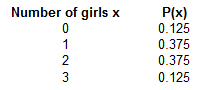The table to the right lists probabilities for the corresponding numbers of girls in three births. What is the random​ variable, what are its possible​ values, and are its values​ numerical?

The random variable is​ x, which is the number of girls in three births. The possible values of x are​ 0, 1,​ 2, and 3. The values of the random value x are numerical.

The variable x is a random variable because its values depend on chance. The possible values of x are​ 0, 1,​ 2, and 3. Because these values are​ numbers, x is a numerical variable.

2

Determine whether the following value is a continuous random​ variable, discrete random​ variable, or not a random variable.

1. The exact time it takes to evaluate 67 + 29
2. The "yes" or "no" response to a survey question
3. The weight of a hamburger
4. The amount of rainfall in a country in a year
5. The time required to upload a file to the Internet
6. The number of people with blood type B in a random sample of 29 people

a. It is a continuous random variable.

b. It is not a random variable.

c. It is a continuous random variable.

d. It is a continuous random variable.

e. It is a continuous random variable.

f. It is a discrete random variable.

3

Determine whether the following value is a continuous random​ variable, discrete random​ variable, or not a random variable.

1. The number of runs scored during a baseball game
2. The last book a person in City A read
3. The number of pigeons in a country
4. The distance a football travels in the air after being thrown
5. The number of hits to a website in a week
6. The number of home runs in a baseball game

a. It is a discrete random variable.

b. It is not a random variable.

c. It is a discrete random variable.

d. It is a continuous random variable.

e. It is a discrete random variable.

f. It is a discrete random variable.

4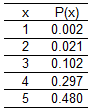Ted is not particularly creative. He uses the pickup line​ "If I could rearrange the​ alphabet, I'd put U and I​ together." The random variable x is the number of girls Ted approaches before encountering one who reacts positively.

Determine whether the table describes a probability distribution. If it​ does, find its mean and standard deviation.

The table is not a probability distribution.

Σ P(x) = 0.902 ≠ 1.00

5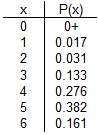Groups of people aged​ 15-65 are randomly selected and arranged in groups of six. In the accompanying​ table, the random variable x is the number in the group who say that their family​ and/or partner contribute most to their happiness​ (based on a​ survey).

If the table is a probability​ distribution, what is its​ mean?

Its mean is 4.5.

Its standard deviation is 1.1.

6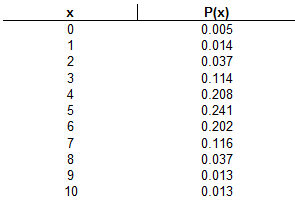The accompanying data table describes results from groups of 10 births from 10 different sets of parents. The random variable x represents the number of girls among 10 children.

Use the range rule of thumb to identify a range of values containing the usual numbers of girls in 10 births.

Based on the​ result, is 1 girl in 10 births an unusually low number of​ girls? Explain.

The maximum usual value is 8.4.

The range rule of thumb for the maximum usual value is given by the formula​ below, where μ and σ are the mean and standard​ deviation, respectively, for a probability distribution.
maximum usual value = μ + 2σ

= 5.034 + 2(1.696126175)

= 8.42625235

The minimum usual value is 1.6.

minimum u sual value = μ – 2σ

= 5.034 – 2(1.696126175)

= 1.64174765

Yes, 1 girl is an unusually low number of​ girls, because 1 girl is outside of the range of usual values.

7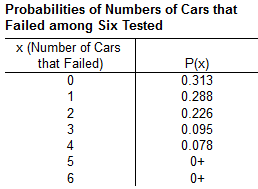The accompanying table describes results of roadworthiness tests of cars that are 3 years old. The random variable x represents the numbers of cars that failed among six that were tested for roadworthiness.

Find the mean and standard deviation for the number of cars that failed among the six cars that are tested.

μ = 1.3

σ = 1.2

8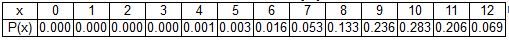Assume that 12 jurors are selected from a population in which 80​% of the people are​ Mexican-Americans. The random variable x is the number of​ Mexican-Americans on the jury.

1. Find the probability of exactly 8 ​Mexican-Americans among 12 jurors.
2. Find the probability of 8 or fewer​ Mexican-Americans among 12 jurors.
3. Which probability is relevant for determining whether 8 jurors among 12 is unusually​ low: the result from part​ (a) or part​ (b)?
4. Is 8 an unusually low number of​ Mexican-Americans among 12 ​jurors? Why or why​ not?

a. P(8​) = 0.133

b. The probability of 8 or fewer​ Mexican-Americans among 12 jurors is 0.206.

(0 + 0 + 0 + 0 + 0.001 + 0.003 + 0.016 + 0.053 + 0.133) = 0.206

c. The result from part​ (b), because it measures the probability of 8 or fewer successes.

d. No, because the relevant probability is greater than 0.05.

9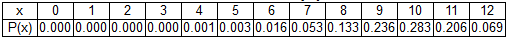Assume that 12 jurors are selected from a population in which 80​% of the people are​ Mexican-Americans. The random variable x is the number of​ Mexican-Americans on the jury.

1. Find the probability of exactly 5 ​Mexican-Americans among 12 jurors.
2. Find the probability of 5 or fewer​ Mexican-Americans among 12 jurors.
3. Which probability is relevant for determining whether 5 jurors among 12 is unusually​ low: the result from part​ (a) or part​ (b)?
4. Is 5 an unusually low number of​ Mexican-Americans among 12 ​jurors? Why or why​ not?

a. P(5​) = 0.003

b. The probability of 8 or fewer​ Mexican-Americans among 12 jurors is 0.004.

(0 + 0 + 0 + 0 + 0.001 + 0.003) = 0.004

c. The result from part​ (b), because it measures the probability of 8 or fewer successes.

d. Yes, because the relevant probability is less than or equal to 0.05.

10

A Gallup poll of 1236 adults showed that​ 12% of the respondents believe that it is bad luck to walk under a ladder. Consider the probability that among 30 randomly selected people from the 1236 who were​ polled, there are at least 2 who have that belief. Given that the subjects surveyed were selected without​ replacement, the events are not independent.

Can the probability be found by using the binomial probability​ formula? Why or why​ not?

Yes. Although the selections are not​ independent, they can be treated as being independent by applying the​ 5% guideline.

Only 30 people are randomly selected from the group of​ 1236, which is less than​ 5%.

11

Determine whether the following probability experiment represents a binomial experiment and explain the reason for your answer.

An experimental drug is administered to 120 randomly selected​ individuals, with the number of individuals responding favorably recorded.

Yes, because the experiment satisfies all the criteria for a binomial experiment.

12

Determine whether the following probability experiment represents a binomial experiment and explain the reason for your answer.

Four cards are selected from a standard​ 52-card deck without replacement. The number of clubs selected is recorded.

No, because the trials of the experiment are not independent and the probability of success differs from trial to trial.

13

​Multiple-choice questions each have five possible answers (a, b, c, d, e)​, one of which is correct. Assume that you guess the answers to three such questions.

1. Use the multiplication rule to find ​P(CWW​), where C denotes a correct answer and W denotes a wrong answer.

a. P(CWW​) = 0.128

P(CWW) = P(C​) x P(W​) x P(W​)

= (0.20) x (0.80) x (0.80) = 0.128

b. ​P(WWC​) = 0.128 P(WCW​) = 0.128

P(WWC) = P(W) x P(W) x P(C)

= 0.80 x 0.80 x 0.20 = 0.128

P(WCW) = P(W) x P(C) x P(W)

= 0.80 x 0.80 x 0.20 = 0.128

c. 0.384

P(CWW) + P(WWC) + P(WCW)

= 0.128 + 0.128 + 0.128

= 0.384

14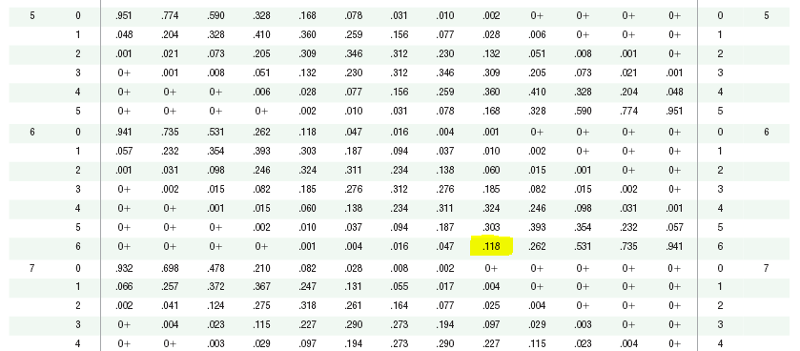Assume that a procedure yields a binomial distribution with 6 trials and a probability of success of 0.70.

Use a binomial probability table to find the probability that the number of successes is exactly 6.

0.118

n = 6

p = 0.70

x = 6

15

Assume that a procedure yields a binomial distribution with a trial repeated n times. Use the binomial probability formula to find the probability of x successes given the probability p of success on a single trial.

n = 20​, x = 3​, p = 0.25

P(3) = 0.134

P(x=3) = ( n x ) x ( p )x x ( q )(n - x)

= ( 20 3 ) x (0.25)3 x (0.75)17

= 0.1338956152

16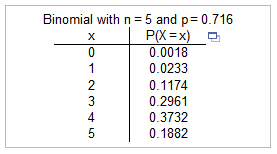Refer to the accompanying technology display. The probabilities were obtained by entering the values of n equals 5 and p equals 0.716. In a clinical test of a​ drug, 71.6​% of the subjects treated with 10 mg of the drug experienced headaches. In each​ case, assume that 5 subjects are randomly selected and treated with 10 mg of the drug.

Find the probability that at least four of the subjects experience headaches.

Is it unusual to have fewer than four subjects experience​ headaches?

The probability that at least four of the subjects experience headaches is 0.5614.

P(x ≥ 4) = P(x=4 or x=5)

= 0.3732 + 0.1882

= 0.5614

No​, because the probability that fewer than four subjects will experience headaches is not unlikely.

17

In a​ region, there is a 0.9 probability chance that a randomly selected person of the population has brown eyes. Assume 10 people are randomly selected.

1. Find the probability that all of the selected people have brown eyes.
2. Find the probability that exactly 9 of the selected people have brown eyes.
3. Find the probability that the number of selected people that have brown eyes is 8 or more.
4. If 10 people are randomly​ selected, is 8 an unusually high number for those with brown​ eyes?

a. The probability that all of the 10 selected people have brown eyes is 0.349.

P(x = 10) = ( 0.910 ) = 0.3486784401

b. The probability that exactly 9 of the selected people have brown eyes is 0.387.

P(x = 9) = ( n x ) x ( p )x x ( q )(n - x)

= ( 10 9) x (0.99) x (0.11)

= 0.387420489

c. The probability that the number of selected people that have brown eyes is 8 or more is 0.930.

P(x = 8) = ( n x ) x ( p )x x ( q )(n - x)

= ( 10 8) x (0.9)8 x (0.1)2

= 0.1937102445

P(x ≥ 8) = P(x=8 or x=9 or x=10)

= 0.194 + 0.387 + 0.349

= 0.930

d. No, because the probability that 8 or more of the selected people have brown eyes is greater than 0.05.

18

Researchers conducted a study to determine whether there were significant differences in graduation rates between medical students admitted through special programs and medical students admitted through the regular admissions criteria. It was found that the graduation rate was 91​% for the medical students admitted through special programs in all medical schools.

1. If 13 of the students admitted through special programs are randomly​ selected, find the probability that at least 12 of them graduated.
2. Suppose a medical school has 13 students in one of the special programs of its medical program. Does the probability calculated in part​ (a) apply to these​ students?

a. The probability that at least 12 of the 13 students graduated is 0.670.

P(x ≥ 12) = P(x=12 or x=13)

= [ ( 13 12 ) x (0.91)12 x (0.09)1 ] + [ (0.9113) ]

= 0.377 + 0.293

= 0.6704

b. No, because the students admitted through a single special program at a specific medical school are not a random sample.

19

When someone buys a ticket for an airline​ flight, there is a 0.0973 probability that the person will not show up for the flight. A certain jet can seat 17 passengers.

Is it wise to book 19 passengers for a flight on the​ jet? Explain.

It is not a wise decision because the probability that there are not enough seats on the jet is 0.436. ​So, overbooking is not an unlikely event.

* n=19, q=0.0973, p=0.9027

P(x > 17) = P(x=18 or x=19)

= [ ( 19 18 ) x (0.9027)18 x (0.0973)1 ] + [ (0.9027)19 ]

= 0.293 + 0.143

= 0.4358

20

The probability of a randomly selected adult in one country being infected with a certain virus is 0.003. In tests for the​ virus, blood samples from 26 people are combined.

What is the probability that the combined sample tests positive for the​ virus?

Is it unlikely for such a combined sample to test​ positive? Note that the combined sample tests positive if at least one person has the virus.

The probability that the combined sample will test positive is 0.075.

P(1 or 2 or ... or 26) = 1 – P(0)

= 1 – [ ( 26 0 ) x (0.0030) x (0.99726) ]

= 1 – 0.925

= 0.0751440049

It is not unlikely for such a combined sample to test​ positive, because the probability that the combined sample will test positive is greater than 0.05.

21

A pharmaceutical company receives large shipments of aspirin tablets. The acceptance sampling plan is to randomly select and test 23 ​tablets, then accept the whole batch if there is only one or none that​ doesn't meet the required specifications.

If a particular shipment of thousands of aspirin tablets actually has a 3​% rate of​ defects, what is the probability that this whole shipment will be​ accepted?

The probability that this whole shipment will be accepted is 0.849.

P(x=0 or x=1)

= [ ( 23 0 ) x (0.03)0 x (0.97)23 ] + [ ( 23 1 ) x (0.03)1 x (0.97)22 ]

= 0.496 + 0.353

= 0.849

22

A pharmaceutical company receives large shipments of aspirin tablets. The acceptance sampling plan is to randomly select and test 19 tablets. The entire shipment is accepted if at most 2 tablets do not meet the required specifications.

If a particular shipment of thousands of aspirin tablets actually has a 3.0​% rate of​ defects, what is the probability that this whole shipment will be​ accepted?

The probability that this whole shipment will be accepted is 0.982.

P(x ≤ 2) = P(x=0 or x=1 or x=2)

= [ (0.97)19 ] + [ ( 19 1 ) x (0.03)1 x (0.97)18 ] + [ ( 19 2 ) x (0.03)2 x (0.97)17 ]

= 0.5606 + 0.3294 + 0.0917

= 0.9817

23

Which of the following is not a requirement of the binomial probability​ distribution?

1. The trials must be dependent.
2. The procedure has a fixed number of trials.
3. The probability of a success remains the same in all trials.
4. Each trial must have all outcomes classified into two categories.

The trials must be dependent.

​"The trials must be​ dependent" is not a requirement of a binomial probability distribution. For a binomial​ distribution, the trials must be independent.

24

Which of the following is NOT one of the three methods for finding binomial probabilities that is found in the chapter on discrete probability​ distributions?

1. Use a simulation
2. Use a statistical table for binomial probabilities
3. Use the binomial probability formula
4. Use computer software or a calculator

Use a simulation

Using a simulation is not one of the suggested methods for finding binomial probabilities.

25

In a clinical trial of a cholesterol​ drug, 427 subjects were given a​ placebo, and 21​% of them developed headaches. For such randomly selected groups of 427 subjects given a​ placebo, identify the values of​ n, p, and q that would be used for finding the mean and standard deviation for the number of subjects who develop headaches.

The value of n is 427.

The value of p is 0.21.

The value of q is 0.79.

26

Assume that a procedure yields a binomial distribution with n trials and the probability of success for one trial is p.

Use the given values of n and p to find the mean μ and standard deviation σ.

Also, use the range rule of thumb to find the minimum usual value μ – 2σ and the maximum usual value μ + 2σ.

n = 1588​, p = 3 / 4

μ = 1191

μ = np

= (1588)(0.75)

= 1191

σ = 17.3

σ = √npq

= √(1588 x 0.75 x 0.25)

= 17.25543393

μ – 2σ = 1156.5

μ + 2 σ = 1225.5

27

Assume that the given procedure yields a binomial distribution with n trials and the probability of success for one trial is p.

Use the given values of n and p to find the mean μ and standard deviation σ.

Also, use the range rule of thumb to find the minimum usual value μ – 2σ and the maximum usual value μ + 2σ.

In an analysis of preliminary test results from the a​ gender-selection method, 19 babies are born and it is assumed that​ 50% of babies are​ girls, so n = 19 and p = 0.5.

The value of the mean is μ = 9.5.

μ = np

= (19)(0.5)

= 9.5

The value of the standard deviation σ = 2.2 .

σ = √npq

= √(19 x 0.5 x 0.5)

= 2.179449472

The minimum usual value is μ – 2σ = 5.1

The maximum usual value is μ + 2σ = 13.9

28

Several psychology students are unprepared for a surprise​ true/false test with 12 ​questions, and all of their answers are guesses.

1. Find the mean and standard deviation for the number of correct answers for such students.
2. Would it be unusual for a student to pass by guessing​ (which requires getting at least 10 correct​ answers)? Why or why​ not?

a. μ = 6

= (12)(0.5)

= 6

σ = 1.7

σ = √npq

= √(12 x 0.5 x 0.5)

= 1.732050808

b. Yes, because 10 is greater than the maximum usual value.

μ – 2σ = 2.6

μ + 2σ = 9.4

29

A headline in a certain newspaper states that​ "most stay at first job less than 2​ years." That headline is based on an online poll of 390 college graduates. Among those​ polled, 76 % stayed at their first​ full-time job less than 2 years.

1. Assuming that​ 50% is the true percentage of graduates who stay at their first job less than two​ years, find the mean and standard deviation of the numbers of such graduates in randomly selected groups of 390 graduates.
2. Assuming that the​ 50% rate in part​ (a) is​ correct, find the range of usual values for the numbers of graduates among 390 who stay at their first job less than two years.
3. Find the actual number of surveyed graduates who stayed at their first job less than two years. Use the range of values from part​ (b) to determine whether that number is unusual. Does the result suggest that the headline is not​ justified?
4. The​ statement, "Alumni who​ opted-in to receive communications from the website were invited to participate in the online​ poll, and 390 of them completed the​ survey," was given as part of the description of the survey methods used. What does that statement suggest about the​ results?

a. The mean is 195 graduates.

μ = np

= (390)(0.50)

= 195

The standard deviation is 9.9 graduates.

σ = √npq

= √(390 x 0.50 x 0.50)

= 9.874208829

b. The numbers of graduates among 390 who stay at their first job less than two years should usually fall between 175.2 and 214.8.

μ – 2σ = 175.2

μ + 2σ = 214.8

c. The actual number of surveyed graduates who stayed at their first job less than two years is 296.

390 x 76% = 296.4 (Round to the nearest whole number)

Since the actual number is outside of the range of usual values found in part​ (b), it is unusual.

The result suggests that the headline is justified.

Because the actual number of surveyed graduates who stayed at their first job less than two years is larger than the maximum usual value under the assumption that​ 50% is the true percentage.​ Therefore, it is unlikely that the true percentage is​ 50% or less.

d. Since the sample of 390 respondents is a voluntary response ​sample, the results are very questionable.

30

A government agency has specialists who analyze the frequencies of letters of the alphabet in an attempt to decipher intercepted messages. In standard English​ text, a particular letter is used at a rate of 6.8​%.

1. Find the mean and standard deviation for the number of times this letter will be found on a typical page of 1600 characters.
2. In an intercepted​ message, a page of 1600 characters is found to have the letter occurring 116 times. Is this​ unusual?

a. μ = 108.8

μ = np

= (1600)(0.068)

= 108.8

σ = 10.1

σ = √npq

= √(1600 x 0.068 x 0.932)

= 10.06983615

b. No, because 116 is within the range of usual values.

μ – 2σ = 88.6

μ + 2σ = 129.0

31

If a gambler places a bet on the number 7 in​ roulette, he or she has a​ 1/38 probability of winning.

1. Find the mean and standard deviation for the number of wins of gamblers who bet on the number 7 one hundred and seventy times.
2. Would 0 wins in one hundred and seventy bets be an unusually low number of​ wins?

a. The value of the mean is μ = 4.5

μ = np

= (170)(1/38)

= 4.473684211

The value of the standard deviation is σ = 2.1

σ = √npq

= √(170 x 1/38 x 37/38)

= 2.087092638

b. Yes, because 0 is below the minimum usual value.

μ – 2σ = 0.3

μ + 2σ = 8.7

32
1. For classes of 80 ​students, find the mean and standard deviation for the number born on the 4th of July. Ignore leap years.
2. For a class of 80 ​students, would two be an unusually high number who were born on the 4th of​ July?

a. The value of the mean is μ = 0.219178

μ = np

= (80)(1/365)

= 0.2191780822

The value of the standard deviation is σ = 0.467523

σ = √npq

= √(80 x 1/365 x 364/365)

= 0.4675228276

b. Yes, because 2 is greater than the maximum usual value.

μ – 2σ = -0.715868 = 0

μ + 2σ = 1.154224

33

For the binomial​ distribution, which formula finds the standard​ deviation?

√npq

The formula √npq is the formula that finds the standard deviation for the binomial distribution.

34

Identify the expression for calculating the mean of a binomial distribution.

np

The formula μ = np is the expression that is equal to the mean of a binomial probability distribution.